• 参数估计matlab函数表 参数估计函数表 函数名 调用形式 函数说明 binofit PHAT= binofit(X, N) [PHAT, PCI] = binofit(X,N) [PHAT, PCI]= binofit (X, N, ALPHA) 二项分布的概率的最大似然估计 置信度为 95%的参数...参数估计matlab函数表

参数估计函数表 函数名 调用形式 函数说明 binofit PHAT= binofit(X, N) [PHAT, PCI] = binofit(X,N) [PHAT, PCI]= binofit (X, N, ALPHA) 二项分布的概率的最大似然估计 置信度为 95%的参数估计和置信区间 返回水平 α 的参数估计和置信区间 poissfit Lambdahat=poissfit(X) [Lambdahat, Lambdaci] = poissfit(X) [Lambdahat, Lambdaci]= poissfit (X, ALPHA) 泊松分布的参数的最大似然估计 置信度为 95%的参数估计和置信区间 返回水平 α 的 λ 参数和置信区间 normfit [muhat,sigmahat,muci,sigmaci] =normfit(X) [muhat,sigmahat,muci,sigmaci] = normfit(X, ALPHA) 正态分布的最大似然估计，置信度为 95% 的置信区间 返回水平 α 的期望、方差值和置信区间 betafit PHAT =betafit (X) [PHAT, PCI]= betafit (X, ALPHA) 返回 β 分布参数 a 和 b 的最大似然估计 返回最大似然估计值和水平 α 的置信区间 unifit [ahat,bhat] = unifit(X) [ahat,bhat,ACI,BCI] = unifit(X) [ahat,bhat,ACI,BCI]=unifit(X, ALPHA) 均匀分布参数的最大似然估计 置信度为 95%的参数估计和置信区间 水平 α 的参数估计和置信区间 expfit muhat =expfit(X) [muhat,muci] = expfit(X) [muhat,muci] = expfit(X,alpha) 指数分布参数的最大似然估计 置信度为 95%的参数估计和置信区间 水平 α 的参数估计和置信区间 gamfit phat =gamfit(X) [phat,pci] = gamfit(X) [phat,pci] = gamfit(X,alpha) γ 分布参数的最大似然估计 置信度为 95%的参数估计和置信区间 最大似然估计值和水平 α 的置信区间 weibfit phat = weibfit(X) [phat,pci] = weibfit(X) [phat,pci] = weibfit(X,alpha) 韦伯分布参数的最大似然估计 置信度为 95%的参数估计和置信区间 返回水平 α 的参数估计及其区间估计 Mle phat = mle( dist ,data) [phat,pci] = mle( dist ,data) 分布函数名为 dist 的最大似然估计 置信度为 95%的参数估计和置信区间 [phat,pci] = mle( dist ,data,alpha) [phat,pci] = mle( dist ,data,alpha,p1) 返回水平 α 的最大似然估计值和置信区间 仅用于二项分布，pl 为试验总次数说明 各函数返回已给数据向量 X 的参数最大似然估计值和置信度为(1- α)×100%的置信区间。α 的默认值为 0.05，即置信度为 95% 。

展开全文• 参数估计包含两种常用方式: 点估计和区间估计.Matlab统计工具箱给出了常用概率分布中参数的点估计 (采用最大似然估计法) 与区间估计,另外还提供了部分分布的对数似然函数的计算功能.由于点估计中的矩估计法的实质是...

参数估计包含两种常用方式: 点估计和区间估计.

Matlab统计工具箱给出了常用概率分布中参数的点估计 (采用最大似然估计法) 与区间估计,

另外还提供了部分分布的对数似然函数的计算功能.

由于点估计中的矩估计法的实质是求与未知参数相应的样本的各阶矩, 统计工具箱提供了常用的求矩函数(见第一章),

读者可根据需要选择合适的矩函数进行点估计.

表2.1　统计工具箱中的参数估计函数 (fit / like)

函数名称

函数说明

调用格式

unifit

均匀分布数据的参数点估计和区间估计

[ahat,bhat,ACI,BCI] = unifit(X,alpha)

expfit

指数分布数据的参数点估计和区间估计

[muhat,muci] = expfit(x,alpha)

normfit

正态分布数据的参数点估计和区间估计

[muhat,sigmahat,muci,sigmaci]

= normfit(X,alpha)

binofit

二项分布数据的参数点估计和区间估计

[phat,pci] = binofit(x,n,alpha)

poissfit

泊松分布数据的参数点估计和区间估计

[lambdahat,lambdaci]

= poissfit(X,alpha)

说明: 调用格式只罗列了其中的一种. 需另外说明的是:

(1) unifit和normfit的格式与其它函数均不同, 此二者要求左边的输出变量必须将参数 或 分别列出.

(2) binofit (x,n,alpha)根据试验成功的次数x和总的试验次数n, 对 中的p进行最大似然估计,

同时返回置信度为100(1-alpha)%的置信区间pci.

【例2-1】(书P692.3) 使用一测量仪器对同一值进行了12次独立测量, 其结果为 (单位:

mm)

232.50, 232.48, 232.15, 232.52, 232.53, 232.30, 232.48, 232.05, 232.45, 232.60, 232.47, 232.30

试用矩法估计测量的真值和方差 (设仪器无系统误差).

·编写命令文件exercise2_3.m：

%P66_2.3 mu与sigma^2的矩估计

x=[232.50, 232.48, 232.15, 232.52, 232.53, 232.30,...

232.48, 232.05, 232.45, 232.60, 232.47, 232.30];

mu_ju=mean(x)

sigma2_ju=var(x,1)

·运行命令文件exercise2_3.m：

>> exercise2_3

mu_ju = 232.4025

sigma2_ju

= 0.0255

【例2-2】(书P692.22) 随机地从一批零件中抽取16个, 测得长度 (单位: cm) 为:

2.14, 2.10, 2.13, 2.15, 2.13, 2.12, 2.13, 2.10,

2.15, 2.12, 2.14, 2.10, 2.13, 2.11, 2.14, 2.11

设零件长度的分布为正态的, 试求总体均值的90%的置信区间:

(1)若

(cm); (2) 若 未知.

(1)·编写函数文件zestimate.m：

%P69_2.22(1)sigma已知时mu的区间估计

function muci=zestimate(x,sigma,alpha)

n=length(x);

xhat=mean(x);

u_alpha=norminv(1-alpha/2,0,1);

delta1=sigma/sqrt(n)*u_alpha;

muci=[xhat-delta1,xhat+delta1];

·调用函数文件zestimate.m：

>> x=[2.14, 2.10, 2.13, 2.15,

2.13, 2.12, 2.13, 2.10, 2.15, 2.12, 2.14, 2.10, 2.13, 2.11, 2.14,

2.11];

>> sigma=0.01;

>> alpha=0.1;

>>

muci=zestimate(x,sigma,alpha)

muci = 2.1209 2.1291

(2)·编写命令文件exercise2_22_2.m：

%P69_2.22(1)sigma未知时mu的区间估计

x=[2.14, 2.10, 2.13, 2.15, 2.13, 2.12, 2.13, 2.10, 2.15, 2.12,

2.14, 2.10, 2.13, 2.11, 2.14, 2.11];

alpha=0.1;

[muhat,sigmahat,muci,sigmaci]= normfit(x,alpha);

muci

·运行命令文件exercise2_22_2.m：

>> exercise2_22_2

muci = 2.1175 2.1325

【例2-3】(书P66例2.31) 对一批产品, 欲通过抽样检查其合格率. 若产品不合格率在5%以下,

则该批产品可出厂. 检验时要求结果具有0.95的置信水平. 今抽取产品100件, 发现不合格品有4件, 问这批产品能否出厂?

>>

[phat,pci]=binofit(4,100,0.05)

phat = 0.0400

pci = 0.0110 0.0993

由于置信区间的上限超出了规定指标(不合格率在5%以下), 因此不能出厂.

展开全文• 在此背景下，采用非参数估计，即不对概率密度函数的形式作任何假设，而是直接用样本估计出整个函数。当然，这种估计只能用数值方法取得，无法得到完美的封闭函数形式。 从另一个角度来看，概率密度函数的参数估计...

非参数估计的基本原理与直方图方法

1. 前言

在很多情况下，我们对样本的分布并没有充分的了解，无法事先给出密度函数的形式，而且有些样本分布的情况也很难用简单的函数来描述。
在此背景下，采用非参数估计，即不对概率密度函数的形式作任何假设，而是直接用样本估计出整个函数。当然，这种估计只能用数值方法取得，无法得到完美的封闭函数形式。
从另一个角度来看，概率密度函数的参数估计实际是在指定的一类函数中选择一个函数作为对未知函数的估计，而非参数估计则可以是看作是从所有可能的函数中进行的一种选择。

2. 直方图方法

非参数概率密度估计的最简单方法：

1. 把样本 x x 的每个分量在其取值范围内分成 k k 个等间隔的小窗。如果 x x d d 维向量，则这种分割就会得到 k d k^d 个小体积或者小舱，每个小舱的体积记作 V V
2. 统计落入每个小舱内的样本数目 q i q_i
3. 把每个小舱内的概率密度看作是常数，并用 q i N V \frac{q_i}{NV} 作为其估计值，其中 N N 为样本总数。3. 非参数估计的基本原理

已知样本集 X = { x 1 , . . . , x N } X=\{x_1,...,x_N\} 中的样本是从服从密度函数 ρ ( x ) \rho(x) 的总体中独立抽取出来的，求 ρ ( x ) \rho(x) 得估计 ρ ( x ) ^ \hat{\rho(x)} 。与参数估计相同，这里不考虑类别，即假设样本都是来自同一个类别，对不同类别只需要分别进行估计即可。
考虑在样本所在空间得某个小区域 R R ，某个随机向量落入这个小区域得概率是：
P R = ∫ R ρ ( x ) d x (1) P_R=\int_{R} \rho(x) dx \tag 1
根据二项分布，在样本集 X X 中恰好有 k k 个落入小区域 R R 得概率是：
P R = C N k P R k ( 1 − P R ) N − k (2) P_R=C_N^k P_R^k(1-P_R)^{N-k} \tag 2
其中 C N k C_N^k 表示在 N N 个样本中取 k k 个的组合数。 k k 的期望值是：
E [ k ] = N P R (3) E[k]=NP_R \tag 3
而且 k k 的众数（概率最大的取值）是：
m = [ ( N + 1 ) P R ] (4) m=[(N+1)P_R] \tag 4
其中 [   ] [ \ ] 表示取整数。因此，当小区域中实际落入了 k k 个样本时， P R P_R 的一个很好的估计是：
P R ^ = k N (5) \hat{P_R} = \frac{k}{N} \tag 5
ρ ( x ) \rho(x) 连续、且小区域 R R 的体积 V V 足够小时，可以假定在该小区域范围内 ρ ( x ) \rho(x) 是常数，则式 ( 2 ) (2) 可近似为：
P R = ∫ R ρ ( x ) d x = ρ ( x ) V (6) P_R=\int_R \rho(x) dx = \rho(x) V \tag 6
用式 ( 5 ) (5) 代入 ( 6 ) (6) ，可得在小区域 R R 的范围内：
ρ ( x ) ^ = k N V (7) \hat{\rho(x)}=\frac{k}{NV} \tag 7
这就是在上面的直方图中使用的对小舱内概率密度的估计。

1. 如果小舱选择过大，则假设 ρ ( x ) \rho(x) 在小舱内为常数的做法就显得粗糙，导致最终估计出的密度函数也非常粗糙；
2. 如果小舱过小，则有些小舱内可能就会没有样本或者很少样本，导致估计出的概率密度函数很不连续。所以，小舱的选择应该与样本总数相适应。理论上讲，假定样本总数是 n n ，小舱的体积为 V n V_n ，在 x x 附近位置上落入小舱的样本个数是 k n k_n ，那么当样本趋于无穷多时 ρ ( x ) ^ \hat{\rho(x)} 收敛于 ρ ( x ) \rho(x) 的条件是：
( 1 ) V n = 0 ( n → ∞ ) ， ( 2 ) k n = ∞ ( n → ∞ ) ， ( 3 ) k n n = 0 ( n → ∞ ) (1) V_n = 0 (n \rightarrow \infty)，(2)k_n = \infty(n \rightarrow \infty)，(3) \frac{k_n}{n} = 0(n \rightarrow \infty)
直观的解释是：随着样本数的增加，小舱体积应该尽可能小(1)，同时又必须保证小舱内有充分多的样本(2)，但每个小舱内的样本数又必须是总样本数中很小的一部分(3)

展开全文非参数估计 直方图 二项分布 概率密度函数估计
• 非参数估计

千次阅读 2020-12-25 22:24:51
出发点：我们以前在参数估计中都是先假设样本点的分布有一个概率密度函数形式，比如高斯分布，然后从样本中估计参数。但是，有可能根本不是，所以也有非参数法研究的必要，比如我们中学就学过频率分布直方图，但是...

引入

出发点：我们以前在参数估计中都是先假设样本点的分布有一个概率密度函数形式，比如高斯分布，然后从样本中估计参数。但是，有可能样本点的分布根本不是高斯分布，那么我们的结果就错了。

本文提出的非参数法讲究不需要先假设样本服从一个什么分布，而是直接从样本中统计得到，比如频率分布直方图。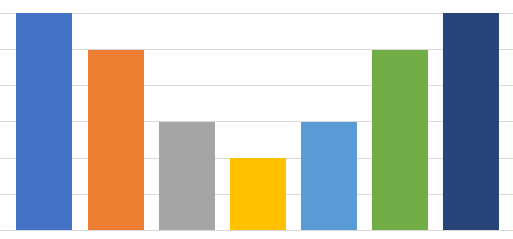这种情况下：我们要记录的是每一组的边界和每一组的数量，然后就能描述我们的样本分布。

理论上，如果我们样本足够多，同时将频率分布直方图组距设置得特别小，组特别多，这就是在逼近样本点的真实概率密度函数。

一般地，样本足够多的时候其可以表示出任意类型的分布！

基础知识

既然非参数法这么好，下面开始介绍基础建筑。这个很好理解，R是指这个一定区域， p ( x ′ ) p(x^{'}) 是概率密度函数。就有点像二维的情况。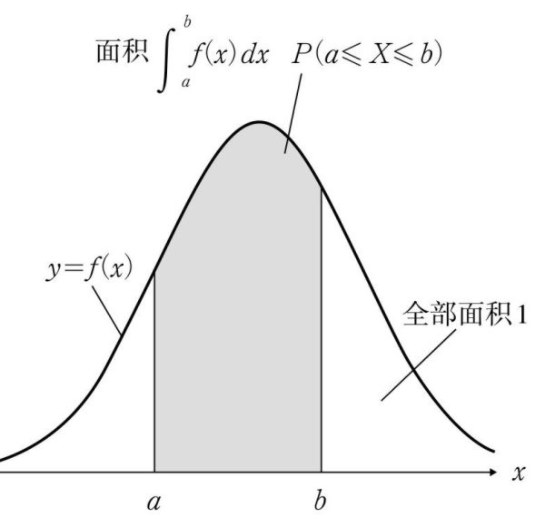所以上上幅图求的是样本落在R空间的概率。
我们继续下一个知识点。该区域等概率密度，那么不就可以算该区域的概率密度了嘛!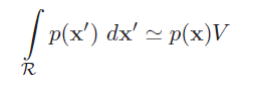而这个由最前面的概念知道，这个是在样本落在空间R中的概率，我们假设R里面有k个样本，整个空间有n个样本，那么样本落在空间R中的概率就是 k n \frac{k}{n}
从而求得空间R中的概率密度函数为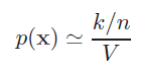我们要估计样本的概率密度函数，但是这个V如何选择呢？这就有点像你要画个频率分布直方图，组距选择多大呢?显然，我们都认为：如果样本数无穷多个，那么我们空间V就选小点，这样就可以表示得更加精确。就像下面这样：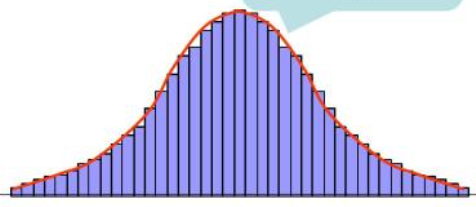因此：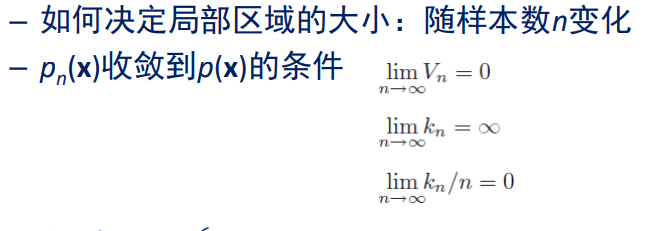解释：上面的 p ( x ) p(x) 表示的是真实概率密度函数，我们希望可以逼近它。
上面都是理论，下面探讨细节。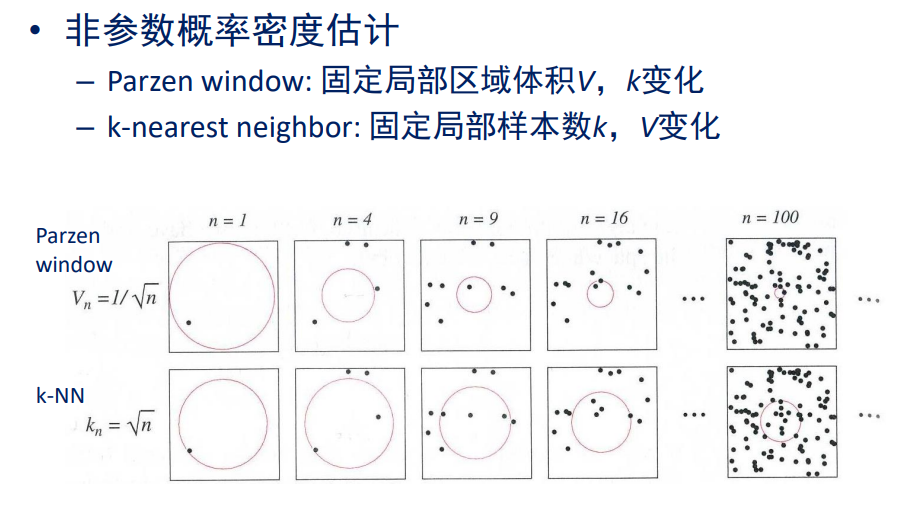上面的大家好好看一下就行，没懂也没关系，后面会展开介绍。

Parzen窗估计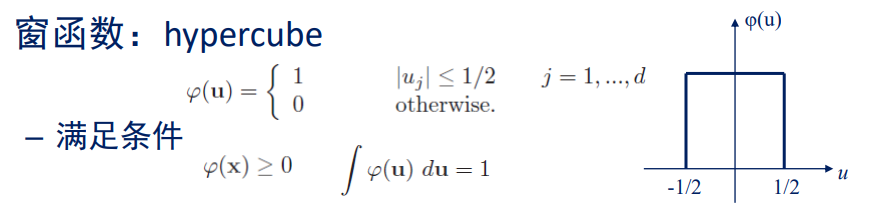解释：这里拓展到了高维的情况，但是低维也适用。样本空间是d维。
然后定义了一个窗函数，即在落在窗口内则函数值为1，否则为0。这个窗口在一维是一个关于原点对称的线段，长度为1；在二维是一个关于原点中心对称的正方形，面积为1；在三维是一个关于原点中心对称的正方体，体积为1。注：上面 h n h_n 是边长。
我们现在用上窗函数了，即对于任意一个样本点 x i x_i ，只要满足向量 x − x i x-x_i 的某一个维度 < = h n / 2 <=h_n/2 ，那么值为1（相当于计数器加1），这不正是我们要求的该区域内样本数吗？
然后代入到之前的公式里。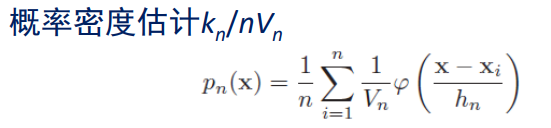我们发现，我们上面用的窗函数是一个超立方体内为1，我们希望推广，找到一些其他的窗函数，但要满足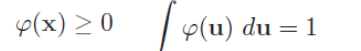即：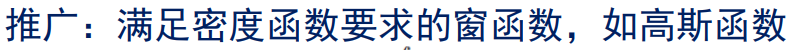为什么窗函数需要满足积分为1？其实主要是因为概率密度函数积分要为1，所以推得窗函数也要。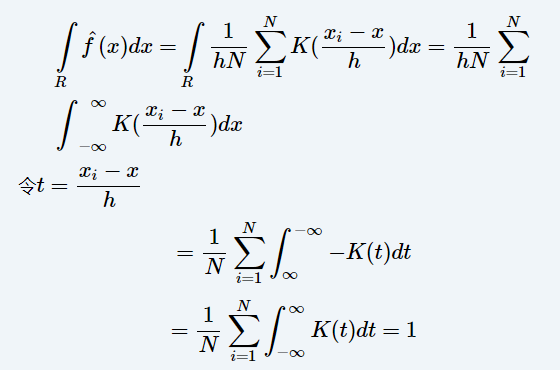除了之前的方窗函数，我们也经常选用如下形式的高斯窗函数。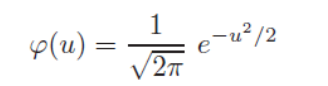然后用这个来估计样本概率密度。（我们先假设1维的情况下）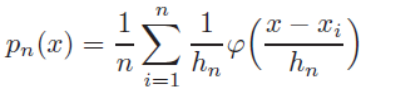例子：下面展示的是只有一个样本点，我们使用高斯窗函数，选用不同的窗口大小得到的估计结果。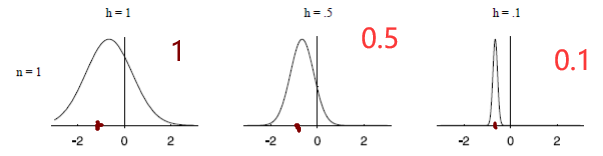请注意，这里的纵轴没有标刻度，实际上 h = 0.1 h=0.1 的时候函数是最高的，但是很窄，方差小。即：例子：
假设有一批样本点，我们选定一个 h n h_n ，有如下结果。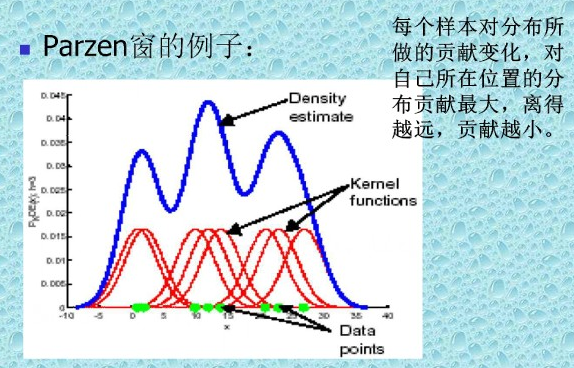解释：
显然，估计的方法是：对每一个样本点的头上套一个窗（红色），然后叠加起来求平均，就是最终的样本概率密度了（蓝色）。（上面展示的图是直接相加，没有求平均，为了好看而已）
显然上面的窗就是下面这个式子（对比 p n ( x ) p_n(x) 的表达式即可）：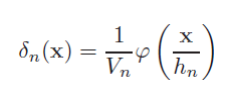注意到，这个式子积分也是为1的！自己可以推一下。
我们知道：直观上样本数量无穷大的时候，然后我们把窗口设得比较小的时候，可以拟合真实的概率密度函数，下面也有一个理论证明：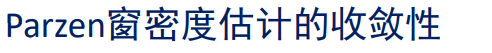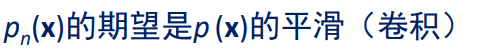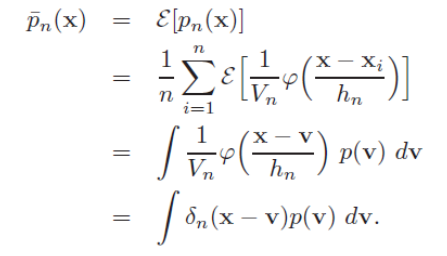上述求和除以n没有了是因为：得到上述的积分等式之后，我们有结论：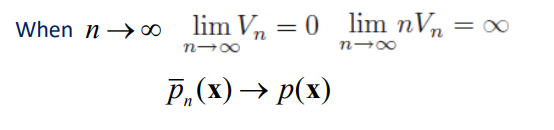我们继续举个分类的例子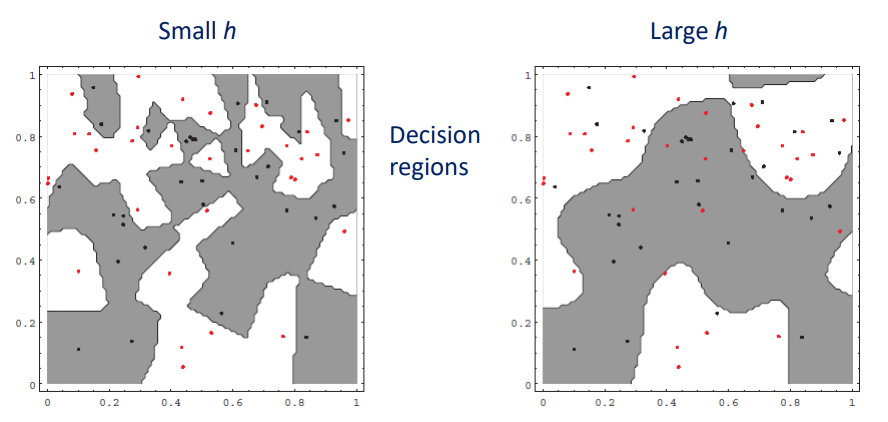使用parzen窗估计做法很简单，就是对每一类拟合一个样本概率密度函数，然后得到分界面如上所示。区别在于，在拟合样本概率密度的时候，选择窗口的大小不同会导致上面的不同，选择小的窗口可以带来过拟合。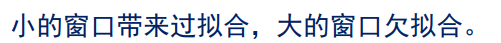改进办法：根据局部密度采用自适应窗口大小。K近邻估计

如何计算样本概率密度？个人觉得V也是一个与k有关的数，k越大，V也越大。
那么如何选择k呢？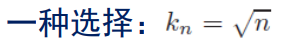例子：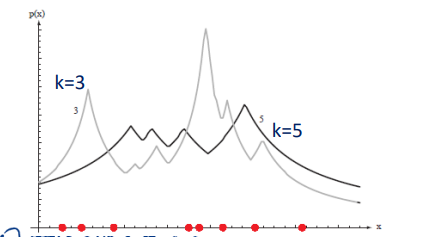有人难以理解，为什么是这样，我们可以假设k=1，那么变成最近邻，在任意样本点 x i x_i 上面，其概率密度函数为 p n ( x i ) = 1 / n 0 = ∞ p_n(x_i)=\frac{1/n}{0}=\infty 。即k越小越突兀。为什么 V n V_n 为0？因为对于 x i x_i 而言，最近的样本点是 x i x_i ，所以，在一维平面上，有 V n = x i − x i = 0 V_n=x_i-x_i=0 。即 V n V_n 是指最近的那个样本点到 x x 的距离的两倍（在一维中是距离或叫线段长度）。
一般地，一维中有：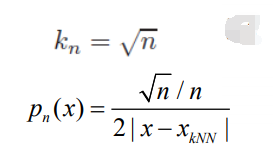x k N N x_{kNN} 是指离 x x 第k个最近的点。
拓展，在二维中 V n V_n 是圆的面积，三维中是球体的体积。
使用KNN来贝叶斯分类：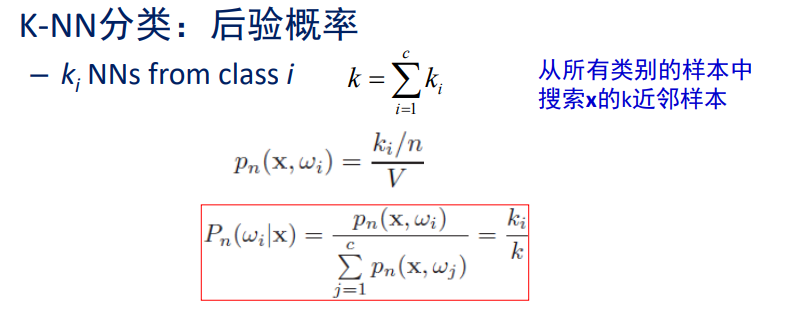非常精彩，红色公式中下面是 p ( x ) p(x) ，上面是 p ( x , w i ) p(x,w_i) 联合概率密度。而且，这和我们的投票方法是一样的， k i k_i 中谁大就分给谁。
当然还有使用KNN分类的办法，上面不是唯一的。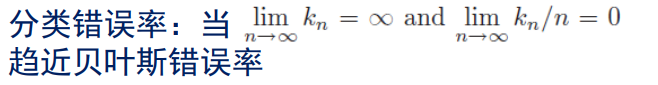原因很简单，因为 n n 趋于无穷的时候， p ( x ) p(x) 估计准确，所以趋近于贝叶斯错误率。
下面介绍一个特殊情况：最近邻分类。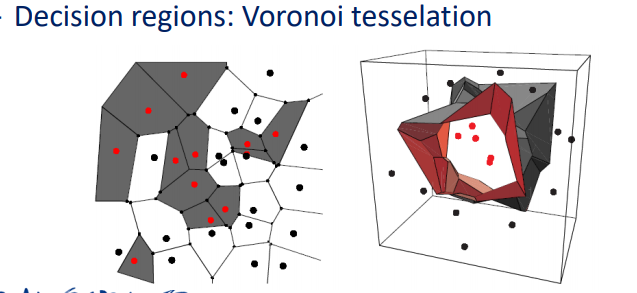K近邻的快速计算

先计算部分欧式距离，总共d维，先计算前r维的距离。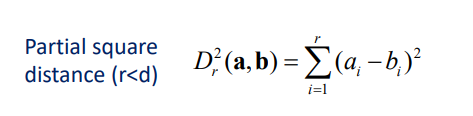假设:我们是快速计算最近邻。
那么快速计算的方法是：先计算x对第一个样本的全部距离，设为最小值，然后计算与第二个样本的部分距离，一旦超过最小值，后面的部分距离就不用算了。
再介绍一个思路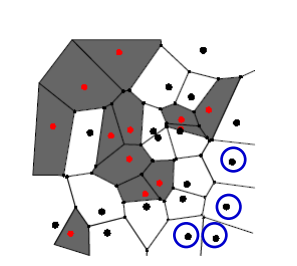即先将样本预处理，将圆形黑色删除，因为删除不会导致误判！

还有一些其他的算法，比如人工智能中很多非常重要的搜索算法，这里可能用得上！总之，应该意识到，快速计算非常重要，否则如果100万个点，k近邻很慢。

Parzen窗方法的快速计算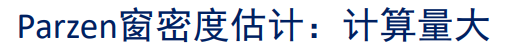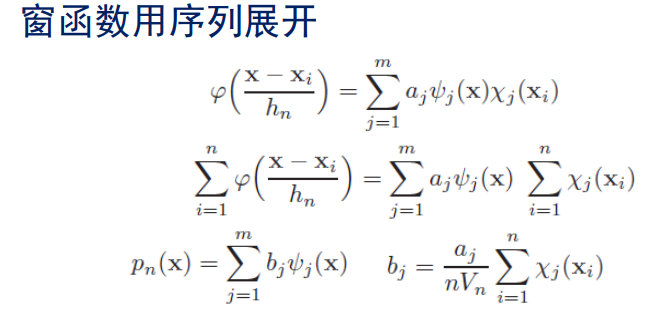其中：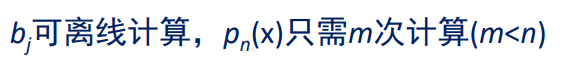这样，预测x的类别的时候就方便了。 b j b_j 早就算好了，算个 p n ( x ) p_n(x) 就行。
例子：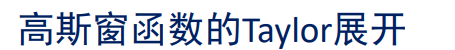展开到第2项。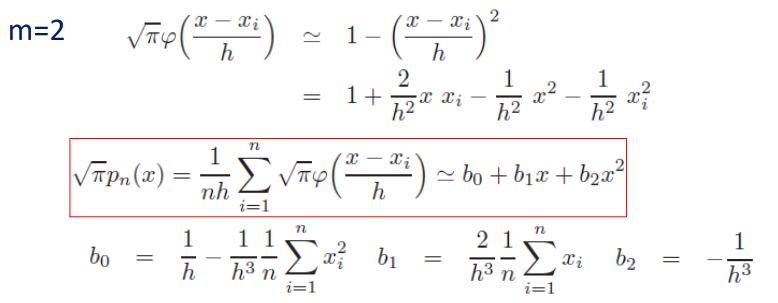从而提前计算好 b 1 , b 2 , b 0 b_1,b_2,b_0 即可。

总结

非参数估计和参数估计有联系但有很大区别，两者都非常重要。前者假定有一个概率密度函数形式，后者可以处理具有任意概率分布形式的数据。
讨论：非参数估计中，在我们对所有训练样本非参数估计后，按理可以得到一个概率密度函数，一般这个是一个具有非常多参数的函数，我们可以保存起来，然后丢弃所有样本，就像在参数估计一样。但是也要注意到，正文中两个快速计算方法都是基于局部空间临时计算概率密度，即所有样本都还要保留，可见这是另一种做法。

展开全文机器学习
• 参数估计 已经知道观测数据符合某些模型的概率下，我们可以利用参数估计的方法来确定这些参数值，然后得出概率密度模型。这个过程中用到了一个条件，就是概率分布符合某些模型这个事实。在这个事实上进行加工。 ...机器学习 python 人工智能 深度学习 java
• 数理统计：参数估计

千次阅读 2020-12-29 22:57:30
learning why, thinking what, then forgetting how. 随着时间的流逝，知识总会被遗忘和...统计推断主要分为参数估计和假设检验，参数估计又分为点估计和区间估计。 2.1 参数的点估计 首先提出参数和参数的估计量的.数理统计 参数估计 信息不等式 均方误差准则
• 最大似然参数估计的基本原理 前导知识：【概率密度函数估计的引入】 在最大似然估计中，我们做以下基本假设： 我们把要估计的参数记作θ\thetaθ，它是确定但未知的量（多个参数时向量）。 每类的样本集记作Xi,i=1,...最大似然参数估计 参数空间 类条件概率密度 最大似然估计量
•   假定一元线性回归方程的具体形式为 y=a+bx(1) y=a+bx \tag{1} y=a+bx(1) 现在，为确定参数a,ba,ba,b进行了nnn次观测，观测结果为： i123⋯nxx1x2x3⋯xnyy1y2y3⋯yn \begin{array}{c|ccccc} i & \text{1} &...一元线性回归方程 参数估计 最小二乘法
• 点击上方“3D视觉工坊”，选择“星标”干货第一时间送达在三维重建中，标定是很重要的一环，而在所有标定中，单目相机标定是最基础的，对于新手而言，跑通了一个相机标定代码，得到了一堆参数结果，...算法 人工智能 计算机视觉 机器学习 编程语言
• 不需要prior参数形式 复杂度取决于训练集的大小或者数据内部的复杂问题 非参数模型也成做实例-based 或者memory-based 学习算法 左右的训练实例存储O（N）的内存，根据所给输入找相似输出的计算量也是O（N），也称作...
• 统计狗来总结一下前面各个楼主的回答，先甩答案：logistic回归模型的参数估计问题，是可以用最小二乘方法的思想进行求解的，但和经典的(或者说用在经典线性回归的参数估计问题)最小二乘法不同，是用的是“迭代重加权...
• ​+β1​ ​x1​+...+βp​ ​xp​exp(β0​ ​+β1​ ​x1​+...+βp​ ​xp​)​ 为了避免异方差，采用加权最小二程的方式获得回归参数估计值 β 0 ^ , β 1 ^ , . . . , β p ^ \widehat{\beta_{0}},\widehat{\...
• R语言中的参数估计

千次阅读 2020-12-25 11:52:44
以下我将从点估计、区间估计来介绍区间估计，本文主要介绍R代码，具体的统计知识，详情可参考相关数理统计的专业书嗷参数估计R语言中的参数估计点估计距估计极大似然估计区间估计均值μ\muμ的估计两样本方差比的...算法
• 介绍参数估计中点估计方法：最大似然估计法，并通过例题加深理解统计学 机器学习
• 介绍参数估计中点估计的常用方法：矩估计法。并通过例题加深理解统计学
• 参数估计问题 在第一课中，提到使用样本估计模型（比如高斯分布）的参数，并说明了常用的极大似然估计法。假设现在有一枚硬币，但它质地不均匀，导致抛硬币的正面朝上与反面朝上的概率不相等，现在还是想研究正面...
• 本发明属于信号处理技术领域，具体涉及一种广义帕累托分布参数估计方法，可用于海杂波背景下的目标检测。背景技术：海杂波背景下的目标检测技术是雷达应用技术中一个至关重要的研究方向，在军事和民用领域已经得到...
• 参数估计方法 概率与统计： 概率：已知模型和参数，推数据结果出现的概率 统计：已知诸多结果，通过结果推概率分布（概率、参数、模型） 比如，我们现在要抛一个硬币，结果会是正面或者反面。我们可以把这个过程，...机器学习 算法 数学
• AR§模型的参数估计 设{XtX_tXt​}适合Xt=a1Xt−1+⋯+apXt−p+ϵtX_t=a_1X_{t-1}+\cdots+a_pX_{t-p}+\epsilon_tXt​=a1​Xt−1​+⋯+ap​Xt−p​+ϵt​,式中{ϵt\epsilon_tϵt​}为独立同分布白噪声序列；Eϵt2=σ2E...python 机器学习 线性代数
• 文章目录一 参数估计二 最大似然估计2.1 参数分量2.2 基本原理2.3 高斯情况2.3.1 协方差矩阵Σ\SigmaΣ已知，而均值μ\muμ未知2.3.2 协方差矩阵Σ\SigmaΣ和均值μ\muμ都未知三 贝叶斯估计3.1 基本原理3.2 高斯...机器学习 人工智能
• 大体上还是遵循着贝叶斯决策论，主要有两个非参数估计的方向： 从训练样本中估计类条件概率密度：p(x∣ωi)p(\textbf{x}|\omega_i)p(x∣ωi​) 直接估计后验概率：P(ωj∣x)P(\omega_j|\textbf{x算法 机器学习
• 基于 MATLAB 的威布尔分布参数估计的图形界面设计 唐军军， 姜年朝， 宋军， 徐艳楠， 刘达 (总参第六十研究所， 江苏 南京 210016) 摘 要： 基于 MATLAB 平台， 设计了一款集最小二乘法、 相关系数法及超概率权重法...
• 白话参数估计

2021-04-21 10:16:10
参数估计是用抽取部分样本的特征，来推断样本总体的特征，举个例子，比如全国人口平均身高，这是个不可能以全体样本来统计的指标，那么就采用抽样的方式，以抽取样本内的平均身高来推断全国的平均身高，为什么说推断...
• 推断统计的工作主要包含两类：参数估计(Parameter Estimation) 和 假设检验(Hypothesis) 。 1.参数估计 参数估计通常有点估计(Point Estimate) 和 区间估计(Interval Estimation) 两种形式。 1.1 点估计 点估计是用...python 统计学 数据分析
• 描述性统计、参数估计和假设检验

万次阅读 多人点赞 2021-04-03 01:15:43
描述性统计分析 描述性统计所提取的统计的信息称为统计量，包括频数与频率，反映集中趋势的均值、中位数、众数和分位数，反映离散程度的极差、方差和标准差，反映分布形状（相对于正态分布）的偏度和峰度。...
• 在机器学习的参数估计（学习）中，常见三种更新方法：梯度下降，极大似然，EM算法；它们的本质目的均是让模型更好地拟合目标分布；在学习三种算法的联系前，需要先了解牛顿法； 牛顿法迭代 先从经典的牛顿法开始，...算法 机器学习
• 估计（随机变量分布的统计推断） 置信区间推断 样本空间：随机变量X构成的空间 参数空间：一个随机变量X的概率密度分布是已知的某种函数形式，该函数与未知参数 有关， 可能是Ω 集中的任意值。称 集为参数空间...
• 还是给你回答了吧parmhat = gpfit(X) 产生pareto随机数参数估计，[parmhat,parmci] = gpfit(X)参数估计，有alpha[parmhat,parmci] = gpfit(X,alpha)GPFIT Parameter estimates and confidence intervals for ......